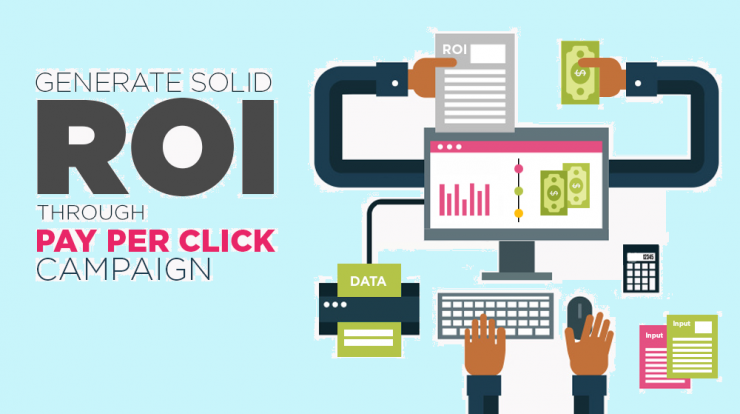Three Ways To Calculate PPC ROI - Genius Question

# Three Ways To Calculate PPC ROIThis is sufficient if you’re collecting leads and not selling goods. You’ll probably end up with more leads in the end. But even if you’re just using PPC(Pay per Click) for lead generation, you should still calculate the return on investment your advertising investment.

So what are the different ways to calculate PPC(Pay per Click) ROI?

Before we get into that, let’s define ROI. It stands for return on investment. The term comes from the finance world and will sound familiar to you if you’ve ever taken accounting. Strictly speaking, ROI is calculated as such:

(Profit – spend) / spend

The sticking point comes in how cost is defined.

### How ROI Works:

• If the average sale increases, so does ROI. If the average sale  decreases, ROI decreases. Ideal situation: The average sale should increase.
• When the cost per click increases, the spend on advertising does too, so the ROI decreases. Ideal situation: Cost per click should decrease.
• When the conversion rate increases, you need to spend less on advertising to get a sale so that the ROI in turn goes up. Ideal situation: Conversion rate should increase.

## Basic ROI Formula and Example

ROI = Net Profit / Total Investment * 100

ROI = 50,000 / 110,000 * 100

ROI = .45 * 100

ROI = 45%

# PPC Management

To get good ROI and better rankings, we at GeniusQuestion implement the following PPC management Process:
•    Complete Website Analysis
•    Keyword Research & Selection